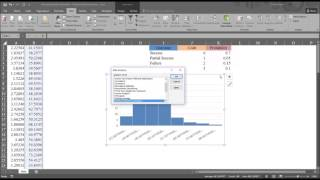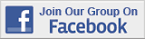Excel Tips & Tutorials:
Random Assignment and Sampling
 Suggest a Link Alphabetize Page Printer-Friendly List
Watch and Learn
Watch and Learn
Watch and Learn
Watch and Learn
 Efficient Data Entry wih Excel FormsLeila Gharani demonstrates (6:21).Random Number Generation with Multiple DistributionsDistributions reviewed include uniform, normal, Bernoulli, binomial, Poisson, patterned, and discrete (11:36).How to Draw Simple and Systematic Random SamplesDemostrated with student population (7:37)Using Excel for Random AssignmentFrom Matthew Lavery (4:31)Efficient Data Entry wih Excel FormsLeila Gharani demonstrates (6:21).Random Number Generation with Multiple DistributionsDistributions reviewed include uniform, normal, Bernoulli, binomial, Poisson, patterned, and discrete (11:36).How to Draw Simple and Systematic Random SamplesDemostrated with student population (7:37)Efficient Data Entry wih Excel FormsLeila Gharani demonstrates (6:21).Random Number Generation with Multiple DistributionsDistributions reviewed include uniform, normal, Bernoulli, binomial, Poisson, patterned, and discrete (11:36).Efficient Data Entry wih Excel FormsLeila Gharani demonstrates (6:21).Sampling with Excel Short how-to article from Real Statistics Using Excel web site.Sampling with Excel Short how-to article from Real Statistics Using Excel web site.Sampling with Excel Short how-to article from Real Statistics Using Excel web site.Sampling with Excel Short how-to article from Real Statistics Using Excel web site.Over 18,000 Members!